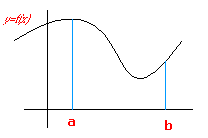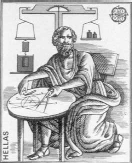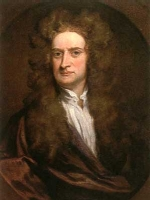2nd year of post-compulsory secondary education ( Natural sciences and Technology). Analysis. INDEX Introduction Aims The method of exhaustion. The definite integral. Properties of the definite integral. The integral function.The definite integral and the area function.
INTRODUCTION

Historically speaking, integral calculus emerged from the need to solve the problem of finding the area of plane figures. It was tackled by the ancient Greeks, who produced formulae to find the area of polygons, the circle and segments of parabolas etc. They used a method which consisted of evaluating the area of a figure exhaustively using polygons of familiar-shaped areas.This method, originally developed by Eudoxus (406 BC - 355 BC), was used sporadically by Euclid (circa 300 BC) and systematically by Archimedes (286 BC - 212 BC).

Around the XVIth century AD this method became known as the method of Exhaustion.Mathematicians of the XVIIth century (Newton, Leibniz etc) based their work on this method and introduced the more general concept of the definite integral of a function, f, on an interval. This concept was later improved on by cauchy (1789-1857) and Reimann (1826-1866).

In this unit we shall demonstrate this method of exhaustion with an example. We shall then apply the same idea to introduce intuitively the concept of the definite integral of a function and we shall focus on some of its properties. Finally, we shall look at the relationship between the definite integral and finding antiderivatives.

AIMS
• To present the concept of the definite integral and its properties visually.
• The aim is not to give rigorous demonstrations but to complement explanations given in the classroom in order to help students assimilate them.

 José Luis Alonso BorregoSpanish Ministry of Education and Education. Year 2001